# formula

(redirected from gait formula)
Also found in: Dictionary, Thesaurus, Medical.

## formula,

in mathematics and physics, equationequation,
in mathematics, a statement, usually written in symbols, that states the equality of two quantities or algebraic expressions, e.g., x+3=5. The quantity x
expressing a definite fixed relationship between certain quantities. The quantities are usually expressed by letters, and their relationship is indicated by algebraic symbols. For example, Ar2 is the formula for the area A of a circle of radius r, and s= 1-2at2 is the formula for the distance s traveled by a body experiencing an acceleration a during a time interval t.

## formula,

in chemistry, an expression showing the chemical composition of a compoundcompound,
in chemistry, a substance composed of atoms of two or more elements in chemical combination, occurring in a fixed, definite proportion and arranged in a fixed, definite structure. A compound is often represented by its chemical formula.
. Formulas of compounds are used in writing the equations (see chemical equationschemical equation,
group of symbols representing a chemical reaction. Basic Notation Used in Equations

The chemical equation 2H2+O2→2H2O represents the reaction of hydrogen and oxygen to form water.
) that represent chemical reactionschemical reaction,
process by which one or more substances may be transformed into one or more new substances. Energy is released or is absorbed, but no loss in total molecular weight occurs.
. Compounds are combinations in fixed proportions of the chemical elementselement,
in chemistry, a substance that cannot be decomposed into simpler substances by chemical means. A substance such as a compound can be decomposed into its constituent elements by means of a chemical reaction, but no further simplification can be achieved.
. The smallest unit of an element is the atomatom
[Gr.,=uncuttable (indivisible)], basic unit of matter; more properly, the smallest unit of a chemical element having the properties of that element. Structure of the Atom
.

### Formulas for Compounds

The formula of a well-known compound, water, is H2O. Water is made up of molecules, and the formula shows that each molecule consists of two atoms of hydrogen, H, bonded to an atom of oxygen, O. The subscript 2 indicates that there are two atoms of hydrogen in the molecule; where no subscript appears, as after the O, the subscript 1 is implied. It should be kept in mind that not all compounds are molecular. For example, sodium chloride, NaCl, is an ionic rather than a molecular compound. Solid sodium chloride consists of a collection of sodium ions and chloride ions arranged in a regular, three-dimensional pattern called a crystalline structure. One cannot say that a certain sodium ion and a certain chloride ion are grouped together into a unit, since each sodium ion is equally associated with all its neighboring chloride ions and each chloride ion is equally associated with all its neighboring sodium ions. The formula NaCl, therefore, cannot be taken as showing the composition of some particular unit, such as a molecule. Rather, it shows the proportion of the atoms of each element making up the compound—in this case, one atom of sodium to every atom of chlorine; such a formula is called an empirical formula.

### Molecular and Empirical Formulas

If a compound is molecular, the molecular formula is preferred to the empirical formula since it gives more information. A molecule of glucose, for example, consists of 6 carbon atoms, 12 hydrogen atoms, and 6 oxygen atoms. Its molecular formula, C6H12O6, displays this information explicitly; the empirical formula is CH2O. From the formula one can also deduce the proportion of the atoms of each element making up the compound: one atom of carbon to every two atoms of hydrogen to every one atom of oxygen (6 : 12 : 6=1 : 2 : 1). The empirical formula of glucose, CH2O, shows only the proportion, not the actual number of atoms.

Many compounds may have the same empirical formula. For example, formaldehyde, each molecule of which consists of one carbon atom, two hydrogen atoms, and one oxygen atom, has the molecular formula CH2O, which is identical to the empirical formula of glucose. Another example is furnished by ethyne (acetylene), whose molecular formula is C2H2, and benzene, whose molecular formula is C6H6. Both have the same empirical formula, CH.

In addition to showing the actual number of atoms, molecular formulas are also more useful than empirical formulas in that they explicitly show radicalsradical,
in chemistry, group of atoms that are joined together in some particular spatial structure and that take part in most chemical reactions as a single unit. Important inorganic radicals include ammonium, NH4; carbonate, CO3 ; chlorate, ClO3
. For example, the molecular formula for the compound aluminum sulfate, Al2(SO4)3, shows that it contains three sulfate radicals (SO4). The empirical formula, Al2S3O12, does not show this. When only one radical is present in the molecule, the parentheses and subscript are omitted, e.g., CuSO4 for cupric sulfate. Other groups are also shown in molecular formulas, e.g., the water molecules in the mineral chalcanthite (blue vitriol), which consists of cupric sulfate atoms to each of which are attached five water molecules. Its molecular formula is CuSO4·5H2O, its empirical formula CuSO9H10.

### Structural Formulas

In many cases, especially with organic compounds, even the molecular formula does not provide enough information to identify a compound, so that structural formulas are needed. For example, both ethanol (ethyl alcohol) and dimethyl ether have the molecular formula C2H6O (see isomerisomer
, in chemistry, one of two or more compounds having the same molecular formula but different structures (arrangements of atoms in the molecule). Isomerism is the occurrence of such compounds. Isomerism was first recognized by J. J. Berzelius in 1827.
). Their structural formulas are: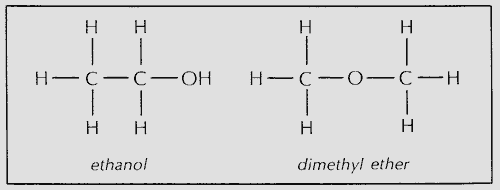In these formulas each line represents a single covalent chemical bondchemical bond,
mechanism whereby atoms combine to form molecules. There is a chemical bond between two atoms or groups of atoms when the forces acting between them are strong enough to lead to the formation of an aggregate with sufficient stability to be regarded as an
. A double bond is represented by a double line and a triple bond by a triple line. In ethene (ethylene), C2H4, the carbon atoms are joined by a double bond. The structural formula of ethene is: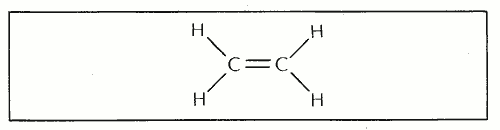(In many representations of structural formulas, the angles of the lines indicating bonds do not necessarily have meaning.) In ethyne (acetylene), C2H2, the carbon atoms are joined by a triple bond. The structural formula of ethyne is: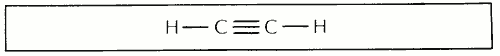#### Semistructural Formulas

Structural formulas are often simplified so that they can be written on a single line; the simplified formulas are often called semistructural formulas. The semistructural formula for ethanol is CH3CH2OH, or more simply C2H5OH. In such a semistructural formula the OH is written explicitly to indicate that the oxygen has a hydrogen bonded to it. The C2H5 indicates that the two carbon atoms are bonded to one another. The semistructural formula for dimethyl ether may be written CH3OCH3. Here the O is placed between the two carbon atoms to show that the carbons are bonded to the oxygen. A carbon often has three hydrogens bonded to it, and the H3 is written after the C. In some cases the H3 is written before the C for clarity; thus the formula for dimethyl ether might be written H3COCH3.

#### Electron Dot Diagrams

Dots are used in a type of formula called the electron dot diagram, where each pair of dots represents a pair of shared electrons in a covalent bond. The diagrams for ethane (CH3CH3), ethene, and ethyne are: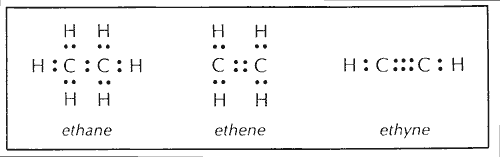The following article is from The Great Soviet Encyclopedia (1979). It might be outdated or ideologically biased.

## Formula

in mathematics, a combination of mathematical symbols that expresses some statement.

Examples of formulas are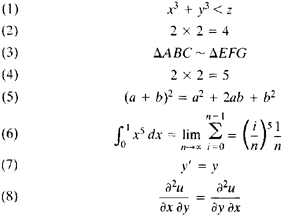It can be seen from these examples that formulas permit rather complicated statements to be written in a compact and convenient form (seeMATHEMATICAL SYMBOLS).

Some formulas—such as (2), (4), and (6) above—express well-defined specific statements and therefore are either true or false. Of these three examples, (2) and (6) are true, and (4) is false.

The sense of other formulas—such as (1), (3), (5), (7), and (8) above—depends on the values of the variables they contain. For example, (1) becomes the true formula 13 + 23 < 19 when x = 1, y = 2, and z = 19; on the other hand, if x = 3, y = 4, and z = 5, we have the false formula 33 + 43 < 5. Formulas of this type may thus be understood as not being intrinsically true or false. Rather, they become true or false when the variables are replaced by specific objects from some previously chosen domain.

Formulas that are true under any replacement of the variables by objects in some domain are said to be identically true in the domain. For example, formula (5) is identically true in the domain of complex numbers, and formula (8) is identically true in the domain of twice continuously differentiable functions of the arguments x and y. Mathematical laws are written by means of formulas that are true, such as (2) and (6), or identically true in some domain, such as (5) and (8). Moreover, identically true formulas are often understood as assertions with universal validity. For example, the most widely held interpretation of formula (5) considers it to be a shorthand form of the assertion “For any numbers a and b the equality (a + b)2 = a2 + 2ab + b2 holds.”

## Formula

in chemistry, a representation of the composition of chemically individual substances by means of chemical symbols and numbers. Chemical formulas generally have the form Am Bn Cp . . . . A, B, C, . . . represent the atoms of the chemical elements that make up a given substance, while m, n, p, . . . are numbers, usually integers, that indicate how many atoms of each element are contained in a given substance. (In the chemical formulas of nonstoichiometric compounds, the numbers may be fractions.)

To determine the formula of a substance it is necessary to find the quantitative composition of the substance in percent by weight, replace the percent content by weight with ratios between the numbers of atoms, and represent these ratios by integers. For example, an analysis of copper pyrite yields 34.64 percent Cu by weight, 30.42 percent Fe, and 34.94 percent S. Dividing these numbers by the atomic weights of Cu (63.55), Fe (55.85), and S (32.06), we obtain 0.545, 0.545, and 1.090, respectively. These numbers have the ratio 1:1:2, from which we obtain the formula for copper pyrite—CuFeS2.

The formulas obtained directly from the results of a quantitative analysis are called empirical, or simplest, formulas. In order to establish the molecular formula of a substance, it is necessary to determine the molecular weight of the substance. If this is not possible, only the empirical formula can be used. The empirical formula contains information only about the quantitative composition of a substance. Molecular formulas include additional information about the actual number of atoms of each element per mole of a substance and, if the substance can be converted into a gas, about the weight of one liter of the gas as well.

Structural formulas represent the interatomic bonds in molecules.

### REFERENCE

Nekrasov, B. V. Osnovy obshchei khimii, vol. 1, 3rd ed. Moscow, 1973.

S. A. POGODIN

## formula

[′fȯr·myə·lə]
(chemistry)
A combination of chemical symbols that expresses a molecule's composition.
A reaction formula showing the interrelationship between reactants and products.
(mathematics)
An equation or rule relating mathematical objects or quantities.
McGraw-Hill Dictionary of Scientific & Technical Terms, 6E, Copyright © 2003 by The McGraw-Hill Companies, Inc.

## formula

1. Maths physics a general relationship, principle, or rule stated, often as an equation, in the form of symbols
2. Chem a representation of molecules, radicals, ions, etc., expressed in the symbols of the atoms of their constituent elements
3.
a. a prescription for making up a medicine, baby's food, etc.
b. a substance prepared according to such a prescription
4. Motor racing the specific category in which a particular type of car competes, judged according to engine size, weight, and fuel capacity
Collins Discovery Encyclopedia, 1st edition © HarperCollins Publishers 2005

## formula

(1)
In logic, a sequence of symbols representing terms, predicates, connectives and quantifiers which is either true or false.

## formula

(language, music)
FORTH Music Language. An extension of FORTH with concurrent note-playing processes. Runs on Macintosh and Atari ST with MIDI output.

["Formula: A Programming Language for Expressive Computer Music", D.P. Anderson et al Computer 24(7):12 (Jul 1991)].

## formula

(3)
Preprocessor language for the Acorn Archimedes, allowing inline high-level statements to be entered in an assembly program. Written in nawk.